Next: Two narrow slits Up: Multiple slits Previous: Multiple slits

## Shortcut

This section focuses on a very useful algebraic simplification we can make to analyze interference patterns. The main lesson is that the intensity at point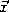is just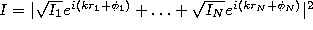, where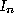is the intensity that slit n would give if it were the only slit,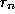is the distance from slit n to the observation point, and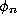is the phase of the waves as they just emerge from slit n. In fact, if you know just this result, you can derive the intensity for any pattern of slits!

To understand this shortcut, we begin with Eqs. 4 & 5, which tell us that the solution coming from the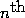slit,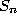, arriving at pointon the screen is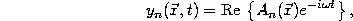with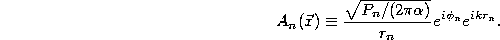The combined solution, therefore, will be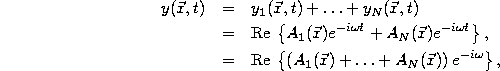which is just simple harmonic motion with a complex amplitude which is the sum of each of the complex amplitudes,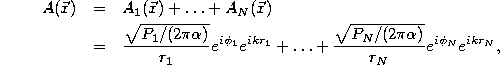where we have used Eq. 7.

We can simplify our work dramatically if we now express everything in terms of the intensities that we would see from each slit if it were the only slit (Eq. 6),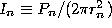. To do this, note that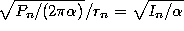. Thus,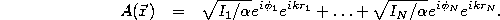Finally, even the factors ofdisappear when we compute the intensity from all of the slits combined,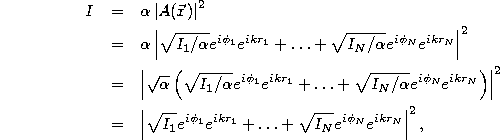which is the simple result we gave at the start of this section. Note that most of this section has been managing algebra to get unknown constant factors to cancel out of the final expression, Eq. 8.

Tomas Arias
Thu Sep 13 15:26:14 EDT 2001